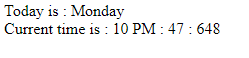# Print current day and time using HTML and JavaScript

• Last Updated : 16 Apr, 2019

The task is to print the system’s current day and time in the following format(Time in hours, minutes and milliseconds).

## Approach:

Use getDay() function to create an array of days in a week. It returns a number of day like 0 for Sunday, 1 for Monday and so on). Convert it into AM or PM using ternary operator.

Use getHours() method to get the hour value between 0 to 23. It returns an integer value.

Minutes and milliseconds were printed using getMinutes() and getMilliseconds() functions respectively.

Example:

 ```<``html``>`` ` `<``head``>``    ``<``title``>``        ``print current day and time``    `````` ` `<``body``>``    ``<``script` `type``=``"text/javascript"``>``        ``var myDate = new Date();``        ``var myDay = myDate.getDay();``       ` `        ``// Array of days.``        ``var weekday = ['Sunday', 'Monday', 'Tuesday',``            ``'Wednesday', 'Thursday', 'Friday', 'Saturday'``        ``];``        ``document.write("Today is : " + weekday[myDay]);``        ``document.write("<``br``/>");``       ` `        ``// get hour value.``        ``var hours = myDate.getHours();``        ``var ampm = hours >= 12 ? 'PM' : 'AM';``        ``hours = hours % 12;``        ``hours = hours ? hours : 12;``        ``var minutes = myDate.getMinutes();``        ``minutes = minutes < ``10` `? '0' + minutes : minutes;``        ``var ``myTime` `= ``hours` `+ " " + ampm + " : " + minutes + ``            ``" : " + myDate.getMilliseconds();``        ``document.write("\tCurrent time is : " + myTime);``    `````` ` ``

## Output:My Personal Notes arrow_drop_up